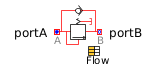Vector Add - MapleSim Help

Sum two vectorsDescription The Vector Add component computes the scaled addition of two vectors.Equations $y=\mathrm{k1}\cdot \mathrm{u1}+\mathrm{k2}\cdot \mathrm{u2}$Connections

 Name Description Modelica ID $\mathrm{u1}$ Real input vector u1 $\mathrm{u2}$ Real input vector u2 $y$ Real output vector yParameters

 Name Default Units Description Modelica ID $\mathrm{k1}$ $1$ Coefficients for ${u}_{1}$ k1 $\mathrm{k2}$ $1$ Coefficients for ${u}_{2}$ k2 $n$ $3$ Dimension of input and output vectors nModelica Standard Library The component described in this topic is from the Modelica Standard Library. To view the original documentation, which includes author and copyright information, click here.